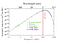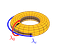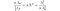# How Planck Spheres Produce the Electron g-factor

Evidence that the universe is made of Planck spheres has been mounting over the past month. The latest discovery involves a core parameter of the electron known as its g-factor. Here I will briefly review the Planck Spherical Unit (PSU) model and then demonstrate how PSUs produce the electron g-factor.

## PSU Review

We begin with the basic assumption that the universe is composed entirely of spheres (PSUs), each with a radius equal to the Planck length ℓP. We find that if the PSUs in the proton volume V have a total mass equal to the Planck mass mP, then the PSUs on the proton surface S will have a total mass equal to the observed proton rest mass mp.

The predicted value for the proton radius rp is 0.8412 fm, which is in perfect agreement with the current CODATA value of 0.8414(19). This basic approach was proposed by Nassim Haramein in 2012 before the proton radius could be accurately measured, so the convergence of measurements around this value represents the first great experimental success of the PSU model.

The next step was to apply a similar approach to the electron. The breakthrough came to me after reading the work of Oliver Consa and David Hestenes who present a convincing case for the electron having a toroidal geometry. In their ring electron models, the electron is a point particle traveling at the speed of light around its Compton wavelength circumference λe. With the circumference of the electron torus known, we can calculate the thickness λ0 necessary for the PSUs on its surface to have a total mass equal to the observed electron rest mass.

T0 is the number of PSUs on the surface of an electron torus with minimum translational velocity (v=0) and thus minimum surface area. The corresponding surface area of an electron torus with maximum translational velocity (v=c) and thus maximum surface area can be expressed as the number of protons that would fit on its surface Tc.

Incredibly, the thickness of the electron torus at rest λ0 is simply a reduction of the proton circumference by a factor of Tc. The fractal nature of this relation is striking on an aesthetic level but the question remained of whether the thickness of this electron torus is a real physical limit. The test is actually straightforward: to detect a photon, it must be transmitted by an electron, and since photons are quantized then the detection of a photon wavelength smaller than the thickness of the electron torus would effectively falsify the model. This predicted minimum photon wavelength (2.0 x 10^-21 m) turns out to be in extremely close agreement with the smallest observed photon wavelength (2.75 x 10^-21 m).

I then turned my attention to the cosmos to determine the relationship between this minimum photon wavelength and the most ubiquitous set of wavelengths in the universe, the cosmic microwave background (CMB). Very simply, the ratio of the electron’s toroidal dimensions of circumference λe to thickness λ0 gives the correct scaling factor between the most intense CMB wavelengths (~1 mm) and the electron Compton wavelength.CMB spectrum

In proportional terms, the minimum photon wavelength λ0 and the most prevalent photon wavelength λb are nearly equidistant from the electron’s Compton wavelength λe. So if the universe was previously condensed into a state with most photons at their minimum wavelength, then we now find ourselves at a point where the dominant photon wavelengths have stretched in size to be nearly equal to λe²/λ0. (Perhaps when the key mysteries of the cosmic scale are resolved, especially the problems of galactic red-shift and galaxy rotation, we’ll find that this represents the steady state condition that the universe advances toward asymptotically.)

## PSU Model of the Electron g-factorPoloidal motion in red and toroidal in blue

Given this proportionality between the dimensions of the electron torus and the CMB, it’s logical to now investigate the same dimensions in relation to the PSU. In discussing the electron’s intrinsic motion we need to make a distinction between the toroidal motion around its circumference λe and the poloidal motion around its thickness, the minimum photon wavelength λ0. As the electron advances along λe, it spirals around λ0 at the speed of light. Since this matches the rate of PSU rotation, the number of PSU rotations in 1 poloidal circuit of λ0 is a simple ratio:

But suppose instead we count the number of PSU rotations as quarter turns of π/2. We can relate this quantity to the number of poloidal circuits equal in length to 1 toroidal circuit by designating a coil of n poloidal circuits as follows:

There is a fractal relationship between the quarter-turn PSU rotations in 1 poloidal circuit and the coils of n poloidal circuits in 1 toroidal circuit. No number other than n generates this simple, self-similar pattern.

We can also calculate the number of quarter-turn PSU rotations in n poloidal circuits and express this new quantity as π*N². This effectively quantifies both the PSU rotations in n poloidal circuits and the squared toroidal-poloidal circuit ratio (reduced by a factor of n) as the area of a circle with radius N.N = 58,567,360

Alternatively, dividing by N we find the number of N PSU quarter turns in n poloidal circuits is simply N units of π.

Now suppose the electron travels N times around λ0 per λe, completing N poloidal circuits per toroidal circuit. Oliver Consa has demonstrated that the toroidal velocity vt of the electron under these conditions is given by the relation:

N is the number of poloidal circuits (a free parameter in his model), r is the poloidal radius (thickness), and R is the toroidal radius (circumference). So applying this to the PSU model proposed here, we find the ratio of the electron’s poloidal velocity c to its toroidal velocity vt is:

Consa identifies this velocity ratio as the “helical g-factor” g. The electron PSU geometry consisting of N, λ0, and λe yields a velocity ratio of 1.00116…, which is half the conventional electron g-factor (2.00232…). Though the precision is limited by the relatively coarse measurement of the proton radius, the agreement between the PSU theoretical value and empirical value leaves little doubt that the model is essentially correct. And the kicker here is that the number n so crucial to these relations is equivalent to the inverse fine-structure constant 1/α with a value of 137.036. Thus for the first time we have a physical explanation for this fundamental constant of nature: it relates the quarter-turn PSU rotations in 1 poloidal circuit to the number of poloidal circuits in 1 toroidal circuit.

From the above relations we see that the factor of (N*λ0/λe)² is equal to α/π.

In QED, the electron g-factor is calculated by an expansion series that begins 1+α/2π+… where the second term is known as the Schwinger factor. Consa’s great insight was to recognize the connection between this factor and the dimensions of the electron torus:

So the electron g-factor is produced by the ratio of the electron’s poloidal velocity to its toroidal velocity. Its poloidal velocity is equal to the speed of light, which is also the velocity of PSU rotation. The number of quarter-turn PSU rotations in 137 poloidal circuits is quantified by the number N, which is the number of poloidal circuits an electron spirals around as it completes 1 toroidal circuit. This yields a velocity ratio (g-factor) in accordance with the Schwinger factor but offers a physical geometric interpretation rather than the opaque set of “renormalization” calculations QED offers. (For more on Consa’s brilliant derivation of the helical g-factor read this paper and for more information about the chicanery involving the QED g-factor then read his paper “Something is Rotten in the State of QED.”)

What should we make of the quarter turn of the PSU that produces the correct value for N? My interpretation at present is that this rotation represents the fundamental PSU deformation. So an electron is nothing more than a quarter turn of the PSU that cascades through successive PSUs along the poloidal-toroidal path laid out here. What makes the quarter turn plausible as a quantum unit of deformation is its ability to efficiently generate dimension. The motion of a point located on the surface of the PSU and oriented along the plane of rotation becomes exactly perpendicular to its origin after a rotation of π/2. The transformation is minimal yet meaningful.

One additional observation about the factor of π/2 is that it is also the ratio of proton Compton wavelength λp to proton radius rp. This may be useful in developing a better understanding of the proton and the “particle zoo” it produces in the aftermath of high energy collisions, though at present I’m unsure of its relevance. Nevertheless, we can express the core distances associated with protons and electrons as a proportionality containing only fundamental constants.

So while string theory regards all of these quantities as essentially random, or totally irrelevant in the case of λ0, the PSU model demonstrates their intrinsic proportionality. It successfully predicts the proton radius. It predicts a minimum photon wavelength in extremely close agreement with observations. It reveals coordination between this minimum photon wavelength and the most prevalent photon wavelengths. Finally, it offers a physical basis for the electron g-factor and reveals the source of the fine-structure constant. Only the correct framework could yield all these results. Our universe consists of harmonic spheres, not chaotic strings, and the paradigm shift has already begun.

[One final note: while I initially agreed with Hestenes’ interpretation of the g-factor as a precession, the new information presented here appears to better fit Consa’s helical solenoid model. So if you go back and read “Protons and Electrons Are Made of Planck Spheres,” which still provides important context for the discussion here, just be aware that I’ve abandoned the precession interpretation in favor of this poloidal-toroidal motion interpretation.]

## More from Matt Lorusso

matt.lorusso@gmail.com